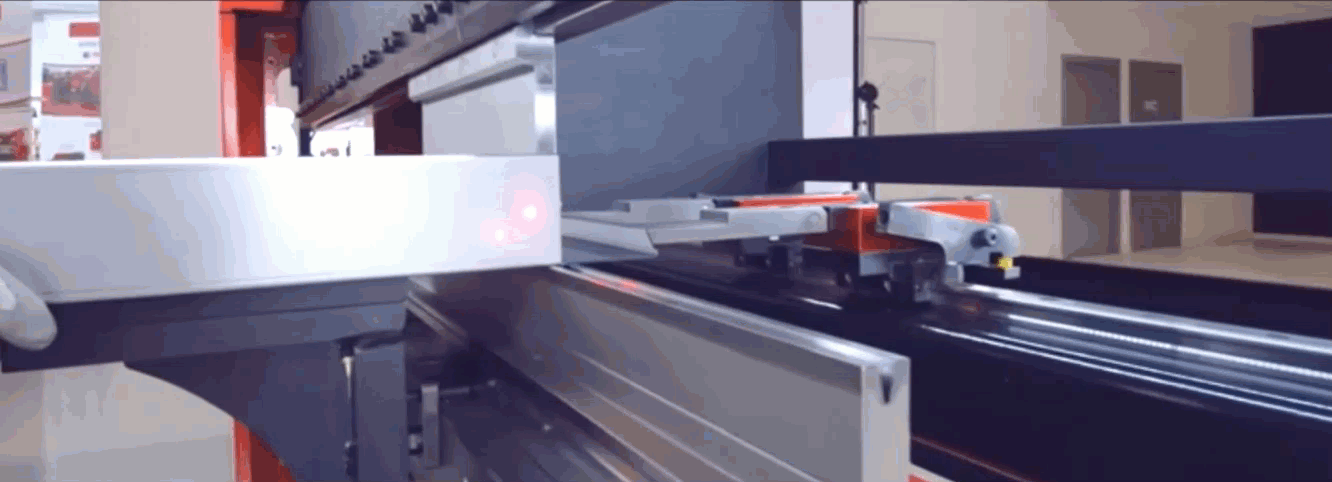# Box Bending

Box, Shelf, Panel, Enclosure whichever way you call them, if you're a fabricator you know what I'm talking about.Those parts with full closure and side legs that hit the ram on the last bend, making us guess:

### How tall of a punch do I need?The solution for this quest comes from ancient Greece... or from high school geometry if you remember it!!

If our box has 90° angles (like 99.9% of boxes do) then the side legs intersecting our punch bending line create a right isosceles triangle (green), and the same bending line creates another right isosceles triangle with the upper beam of our PB (brown).

Either we know how tall our punch is or how tall our side legs need to be. In our example we know:

• H=height of our punch

• h=height of our side leg

• &=45° angle (half of the 90° we're bending our box to)

• W=distance from the bending line to the edge of the ram or clamp hitting the box

Remember Trig rules... SOHCAHTOA.... well, let's just remember the last 3 letters TOA:

Being a right isosceles triangle we know

Tan(&)=1 because a=h (that's why we call them isosceles)

We also know there's a couple direct relations between b and h

b h x 1.4144....

h(or a) = b x 0.707

The same trig rules apply to the brown triangle. which means that

W= C (which is the missing height of our punch once we have bIf we know the height of our enclosure side leg (h) and we want to know the height of the upper tool we need, we run the following operation:

H = (h x 1.4144)+W (h and W are variables we can easily measure)

If we know the height of the punch and want to know the max height of our box side legs:

h = (H - W) x 0.707